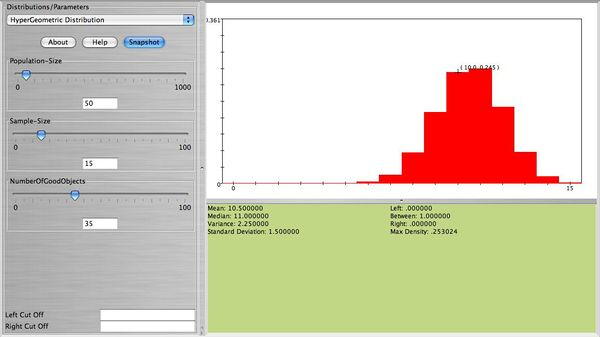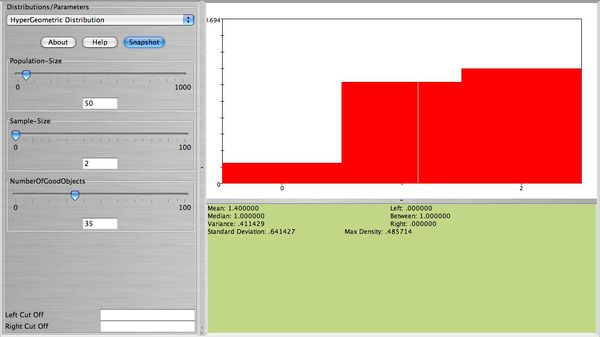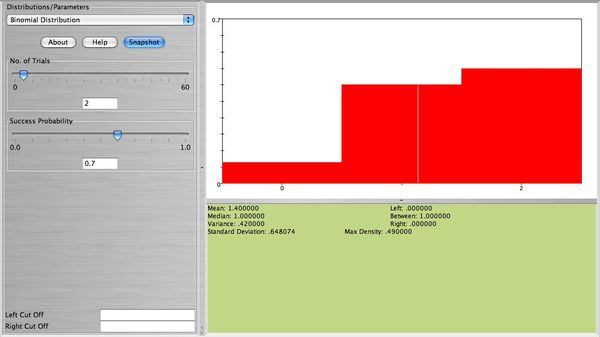# AP Statistics Curriculum 2007 Limits Bin2HyperG

## General Advance-Placement (AP) Statistics Curriculum - Binomial Approximation to HyperGeometric Distribution

### Binomial Approximation to HyperGeometric Distribution

Suppose $$X \sim Hypergeometric(m, N, n)$$ and $$p=m/N$$.

### Examples

An urn contains 50 marbles (35 green and 15 white). Fifteen marbles are selected without replacement. Find the probability that exactly 10 out of the 15 selected are green marbles. The answer to this question can be found using the formula$P(X=10)=\frac{{35 \choose 10}{15 \choose 5}}[[:Template:50 \choose 15]]=0.2449.$ Using SOCR simply enter population size 50, sample size 15, and number of good objects 35, to get the figure below.Now, select without replacement only 2 marbles. Compute the exact probability that 1 green marble is obtained. This is equal to $$P(X=1)=\frac{{35 \choose 1}{15 \choose 1}}[[:Template:50 \choose 2]]=0.4286.$$ This is also shown on the figure below.We will approximate the probability of obtaining 1 green marble using Binomial as follows. Select the SOCR Binomial distribution and choose number of trials 2 and probability of success $$p=\frac{35}{50}=0.7$$. Compare the figure below with the figure above. They are almost the same! Why? Using the Binomial formula we can compute the approximate probability of observing 1 green marble as $$P(X=1)={2 \choose 1}0.70^10.30^1=0.42$$ (very close to the exact probability, 0.4286)."-----